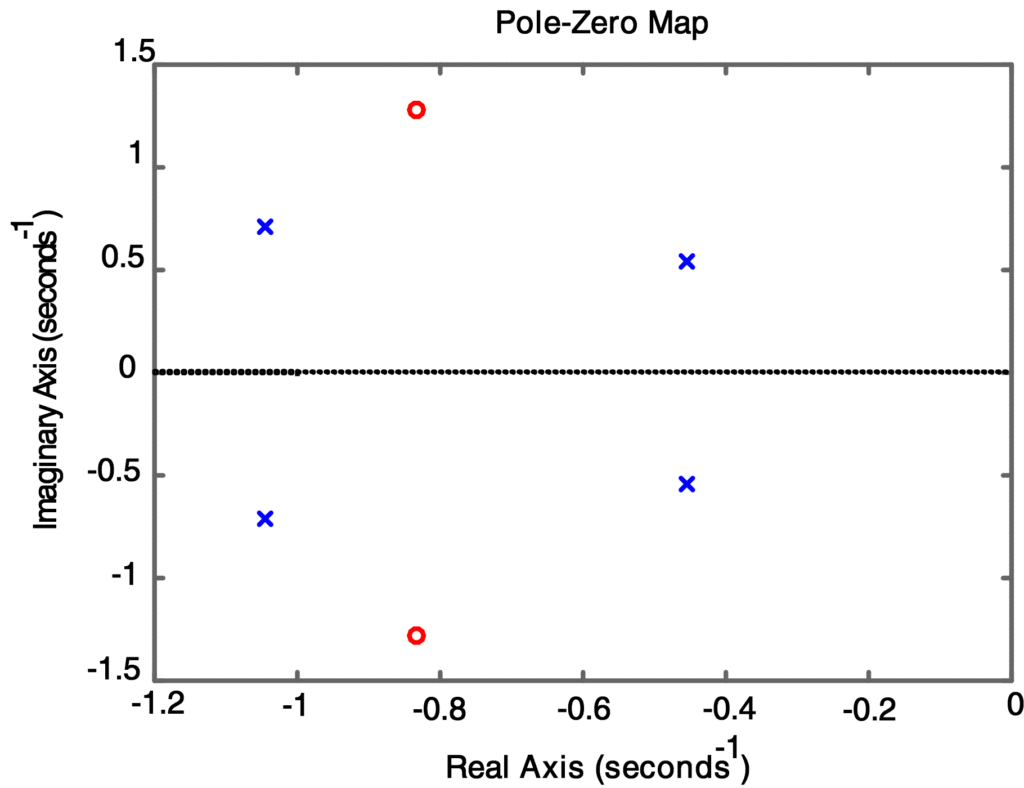Home / Control with Matlab / Transfer Function Calculation Matlab with Example

# Transfer Function Calculation Matlab with Example

Want create site? Find Free WordPress Themes and plugins.

## Transfer Functions Representation

Consider a ﬁxed single-input/single-output linear system with input u(t) and output y(t) given by the differential equation

$\begin{matrix} \overset{..}{\mathop{y}}\,+6\overset{.}{\mathop{y}}\,+5y=4\overset{.}{\mathop{u}}\,+3u & \cdots & (1) \\\end{matrix}$

Applying the Laplace transform to both sides of (1) with zero initial conditions, we obtain the transfer function of the system from the input U (s) to the output Y(s) in TF form as the ratio of polynomials:

$\begin{matrix} G(s)=\frac{4s+3}{{{s}^{2}}+6s+5} & \cdots & (2) \\\end{matrix}$

Alternatively, this transfer function can be expressed in terms of its zeros zi, poles pj, and gain K in the factored, or Zero-Pole (ZP) form, as

$\begin{matrix} G(s)=\frac{4(s+0.75)}{(s+1)(s+5)} & \cdots & (3) \\\end{matrix}$

Which shows that G(s) has a single zero at s = -0.75, two poles at s = -1 and -5, and a gain of 4.

## Transfer Function Using Numerator and Denominator Coefficients

If we know the numerator and denominator polynomials of G(s), we can represent the model in MATLAB by creating a pair of row vectors containing the coefﬁcients of the powers of s, in descending order, of the numerator and denominator polynomials. For the transfer function in (2), we would enter numG = [4 3] and denG = [1 6 5].

If the transfer function is known in terms of its zeros, poles, and gain, we can create the model by entering column vectors for the zeros and poles, and enter the gain as a scalar. Then the model is represented by these three quantities, which can be used as arguments in commands for performing calculations. To create the system described in (3), we would enter the commands zG = -0.75, pG = [-1; -5],and kG = 4.

When a model has been described in MATLAB in either one of these forms, the Control System Toolbox provides several functions to obtain the description in the other form.

## Commands to Create Transfer Functions

For example, if the numerator and denominator polynomials are known as the vectors numG and denG, we merely enter the MATLAB command [zz, pp, kk] = tf2zp (numG, denG). The result will be the three-tuple [zz, pp, kk] , which consists of the values of the zeros, poles, and gain of G(s), respectively. Alternatively, if the zeros, poles, and gain are known as the column vectors zG and pG and the scalar kG, we would enter the command [num, den] = zp2tf (zG, pG, kG) .

## Transfer Function Graphical Representation

Once we have the system model in either the TF or the ZP form, we can obtain a graphical representation of the transfer functions poles and zeros by using the pzmap command from the Control System Toolbox. The two forms of the command that are applicable to transfer-function models are pzmap(numG,denG) and pzmap(pG, zG), where in the ﬁrst case the arguments are row vectors and in the latter case, the arguments are column vectors. In either case, a region of the s-plane is shown with the zeros indicated by “O” and the poles by “X”.

## Transfer Function Matlab Example

Convert G(s) to Factored Form

Find the transfer function in both the polynomial (TF) and factored (ZP) forms for the fourth-order system whose differential equation is

$\overset{….}{\mathop{y}}\,+3\overset{…}{\mathop{y}}\,+4\overset{..}{\mathop{y}}\,+2.5\overset{.}{\mathop{y}}\,+0.8y=3\overset{..}{\mathop{u}}\,+5\overset{.}{\mathop{u}}\,+7u$

Solution

Taking the Laplace transform of the differential equation with zero initial conditions, we obtain the polynomial form of the transfer function as

$G(s)=\frac{3{{s}^{2}}+5s+3}{{{s}^{4}}+3{{s}^{3}}+4{{s}^{2}}+2.5s+0.8}$

The MATLAB commands in Script 1 will create the polynomial form of G(s) and then use it to determine the factored form. From the numerical output we ﬁnd that G(s) has zeros at s = -0.8333± j1.2802, poles at s == -0.4548±j0.5420 and s = -1.0452±j0.7110, and a gain of 3.

## Matlab Code to Find the Transfer Function


% Script 1: Matlab Code to Find the transfer function

%in both the polynomial (Transfer-Function) and factored (Zero-Pole) forms

numG = [3 5 7] % enter transfer function numerator

denG = [1 3 4 2.5 0.8] % ...and denominator polynomials

% convert to factored (ZP) form

[zz,pp,kk] = tf2zp(numG,denG)

pzmap(numG,denG) % pzmap from TF form

pzmap(pp,zz) % pzmap from ZP formDid you find apk for android? You can find new Free Android Games and apps.

### About Ahmad FaizanMr. Ahmed Faizan Sheikh, M.Sc. (USA), Research Fellow (USA), a member of IEEE & CIGRE, is a Fulbright Alumnus and earned his Master’s Degree in Electrical and Power Engineering from Kansas State University, USA.